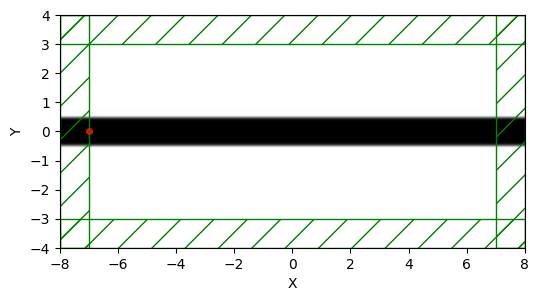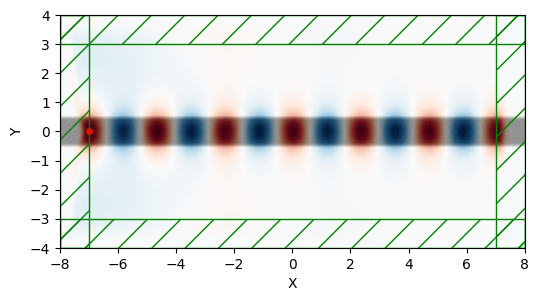# A straight waveguide¶

For our first example, let's examine the field pattern excited by a localized continuous wave (CW) source in a waveguide — first straight, then bent. The waveguide will have frequency-independent $\epsilon$=12 and width 1 $\mu$m. The unit length in this example is 1 $\mu$m. See also Units.

The first thing to do always is to load the Meep library, along with any other library we may need for post processing or visualization:

In :
import meep as mp

Using MPI version 3.1, 1 processes


## Simulation Domain¶

We can begin specifying each of the simulation objects starting with the computational cell.

We're going to put a source at one end and watch the fields propagate down the waveguide in the $x$ direction, so let's use a cell of length 16 $\mu$m in the $x$ direction to give it some distance to propagate. In the $y$ direction, we just need enough room so that the boundaries do not affect the waveguide mode; let's give it a size of 8 $\mu$m.

In :
cell = mp.Vector3(16,8,0)


The Vector3 object stores the size of the cell in each of the three coordinate directions. This is a 2d cell in $x$ and $y$ where the $z$ direction has size 0. All 2D simulations must lie in the XY plane, as we've specified.

## Geometry¶

Next we add the waveguide. Most commonly, the device structure is specified by a set of GeometricObjects stored in the geometry object.

In :
geometry = [mp.Block(mp.Vector3(mp.inf,1,mp.inf),
center=mp.Vector3(),
material=mp.Medium(epsilon=12))]


The waveguide is specified by a Block (parallelepiped) of size $\infty \times 1 \times \infty$, with $\epsilon$=12, centered at (0,0) which is the center of the cell. By default, any place where there are no objects there is air ($\epsilon$=1), although this can be changed by setting the default_material variable (shown below).

## Sources¶

We have the structure and need to specify the current sources using the sources object. The simplest thing is to add a single point source $J_z$:

In :
sources = [mp.Source(mp.ContinuousSource(frequency=0.15),
component=mp.Ez,
center=mp.Vector3(-7,0))]


We gave the source a frequency of 0.15, and specified a ContinuousSource which is just a fixed-frequency sinusoid exp($−i\omega t$) that by default is turned on at $t=0$.

Recall that, in Meep units, frequency is specified in units of $2\pi c$, which is equivalent to the inverse of the vacuum wavelength. Thus, 0.15 corresponds to a vacuum wavelength of about 1/0.15=6.67 μm, or a wavelength of about 2 μm in the ε=12 material — thus, our waveguide is half a wavelength wide, which should hopefully make it single mode. In fact, the cutoff for single-mode behavior in this waveguide is analytically solvable, and corresponds to a frequency of $\frac{1}{2\sqrt{11}}$ or roughly 0.15076.

Note also that to specify a $J_z$, we specify a component Ez (e.g., if we wanted a magnetic current, we would specify Hx, Hy, or Hz).

The current is located at (-7,0), which is 1 $\mu$m to the right of the left edge of the cell — we always want to leave a little space between sources and the cell boundaries, to keep the boundary conditions from interfering with them.

## Boundary layers¶

As for boundary conditions, we want to add absorbing boundaries around our cell.

Absorbing boundaries in Meep are handled by perfectly matched layers (PML) — which aren't really a boundary condition at all, but rather a fictitious absorbing material added around the edges of the cell. To add an absorbing layer of thickness 1 $\mu$m around all sides of the cell, we do:

In :
pml_layers = [mp.PML(1.0)]


pml_layers is a list of PML objects — you may have more than one PML object if you want PML layers only on certain sides of the cell. For example, mp.PML(thickness=1.0,direction=mp.X,side=mp.high) specifies a PML layer on only the +x side.

An important point: the PML layer is inside the cell, overlapping whatever objects you have there. So, in this case our PML overlaps our waveguide, which is what we want so that it will properly absorb waveguide modes.

The finite thickness of the PML is important to reduce numerical reflections. For more information, see Perfectly Matched Layer.

## Resolution¶

Meep will discretize this structure in space and time, and that is specified by a single variable, resolution, that gives the number of pixels per distance unit.

We'll set this resolution to 10 pixels/μm, which corresponds to around 67 pixels/wavelength, or around 20 pixels/wavelength in the high-index material. In general, at least 8 pixels/wavelength in the highest dielectric is a good idea. This will give us a 160×80 cell.

In :
resolution = 10


## Simulation Object¶

The final object to specify is Simulation which is based on all the previously defined objects:

In :
sim = mp.Simulation(cell_size=cell,
boundary_layers=pml_layers,
geometry=geometry,
sources=sources,
resolution=resolution)


## Visualization¶

Before we actually run our simulation, we often want to visualize the entire domain to ensure that the geometry, source, boundary layers, and even monitors are all correct.

We can create a figure using matplotlib, and pass the figure axis to the plot2D function. This function plots all of the relevant simulation objects in the passed axis handle. Any arbitrary slice may be specified. The default is through z=0.

In :
from matplotlib import pyplot as plt
%matplotlib inline
plt.figure(dpi=100)
sim.plot2D()
plt.show()

-----------
Initializing structure...
block, center = (0,0,0)
size (1e+20,1,1e+20)
axes (1,0,0), (0,1,0), (0,0,1)The green boxes represent the PML boundary layers. The black line through the middle is our waveguide. The red dot is our source.

Everything looks like we would expect, so let's run the simulation.

## Simulate¶

We are ready to run the simulation. We time step the fields until a time of 200:

In :
sim.run(until=200)

run 0 finished at t = 200.0 (4000 timesteps)


It should finish in less than a second. We can analyze and visualize the fields using plot2D:

In :
plt.figure(dpi=100)
sim.plot2D(fields=mp.Ez)
plt.show()We can see the Ez component of the fields superimposed on the previous domain plot. We can choose to plot any of the six field components specified earlier.

## Animation¶

Often, we want to track the evolution of the fields as a function of time. This helps us ensure the fields are propogating as we would expect.

We can easily accomplish this using a run function. Run functions are passed to the sim.run() method and can be called every time step. The Animate2D() run function can be used to generate an animation object by grabbing frames from an arbitrary number of time steps.

We need to pass the sim object we created, specify which fields component we are interested in tracking, specify how often we want to record the fields, and whether to plot everything in real time. For this simulation, let's look at the Ez fields and take a snapshot every 1 time units.

Unfortunately, Jupyter notebooks don't render realtime figure updates natively, so we'll turn this feature off and just view the animation after it runs.

We also want to restart the field propogation by calling reset_sim().

In :
sim.reset_meep()
f = plt.figure(dpi=100)
Animate = mp.Animate2D(sim, fields=mp.Ez, f=f, realtime=False, normalize=True)
plt.close()


Once defined, we can pass it along with our original argument, until. This time, however, we'll just run until 50 time units. We'll tell meep to record the field information every 1 time unit by using the at_every() run function modifier.

In :
sim.run(mp.at_every(1,Animate),until=100)
plt.close()

-----------
Initializing structure...
block, center = (0,0,0)
size (1e+20,1,1e+20)
axes (1,0,0), (0,1,0), (0,0,1)
Normalizing field data...
run 1 finished at t = 100.0 (2000 timesteps)


Now that we've run the simulation, we can postprocess the animation and export it to an mp4 video using the to_mp4() method. We'll specify a filename and 10 frames-per-second (fps).

In :
filename = "media/straight_waveguide.mp4"
Animate.to_mp4(10,filename)

Generating MP4...


Finally, we can use some iPython tools to visualize the animation natively.

In :
from IPython.display import Video
Video(filename)

Out:

As expected, the fields propogate down the waveguide.